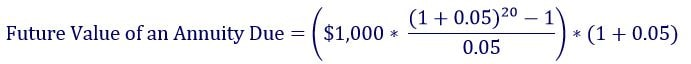#### This video shows how to calculate the future value of an annuity due. An annuity due is a series of periodic cash flows that begin today. Thus, if we were going to deposit \$500 annually in a savings account starting today, we could calculate the balance after 30 years. This is just one example of what it means to calculate the future value of an annuity due. It is different from the future of an ordinary annuity, because with the ordinary annuity you wouldn’t make the first deposit until the end of the first period (with an annuity due, you make the first cash flow today). The video provides a formula to show you how to calculate the future value of an annuity due.

When we calculate the future value of an annuity due, we are figuring out the ending balance of a series of periodic cash flows that begin today.  For example, if we were going to deposit \$500 annually in a savings account with the first deposit being made today, we could compute what the balance would be after 30 years’ time.  The ending balance is the future value of the annuity due.

An annuity due is different from an ordinary annuity, because with the ordinary annuity you wouldn’t make the first deposit until the end of the first period (with an annuity due, you make the first cash flow today).  Here is the formula for calculating the future value of an annuity due:where:

r = the discount rate

n = the number of periods

Cash Flow = the amount of the periodic cash flow

Let’s say you plan to deposit \$1,000 in an investment account every year for 20 years so you can retire and live the good life, backpacking across Australia and reading finance textbooks.  You will make your first deposit of \$1,000 today, and you expect to receive a return of 5%.  To find out how much money you will have at the end of the end of 20 years, we just plug these numbers into the formula above:The calculations above show that you will have \$34,719.25 after 20 years.  I’m not sure if that’s enough money to retire and travel Australia, but it will sure get you a lot of finance textbooks.